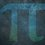# UKMT Special (Problem $13$)

For each positive real integer $x$, we define {$x$} to be the greater of $\frac{1}{x}$ and $x$, with {$1$} $=1$

Find, with proof, all positive real numbers $y$ such that

$5y \{ 8y \} \{ 25y \} = 1$

[UKMT BMO $2016$ Round $1$, Q$2$]Note by Yajat Shamji
8 months, 2 weeks ago

This discussion board is a place to discuss our Daily Challenges and the math and science related to those challenges. Explanations are more than just a solution — they should explain the steps and thinking strategies that you used to obtain the solution. Comments should further the discussion of math and science.

When posting on Brilliant:

• Use the emojis to react to an explanation, whether you're congratulating a job well done , or just really confused .
• Ask specific questions about the challenge or the steps in somebody's explanation. Well-posed questions can add a lot to the discussion, but posting "I don't understand!" doesn't help anyone.
• Try to contribute something new to the discussion, whether it is an extension, generalization or other idea related to the challenge.

MarkdownAppears as
*italics* or _italics_ italics
**bold** or __bold__ bold
- bulleted- list
• bulleted
• list
1. numbered2. list
1. numbered
2. list
Note: you must add a full line of space before and after lists for them to show up correctly
paragraph 1paragraph 2

paragraph 1

paragraph 2

[example link](https://brilliant.org)example link
> This is a quote
This is a quote
    # I indented these lines
# 4 spaces, and now they show
# up as a code block.

print "hello world"
# I indented these lines
# 4 spaces, and now they show
# up as a code block.

print "hello world"
MathAppears as
Remember to wrap math in $$ ... $$ or $ ... $ to ensure proper formatting.
2 \times 3 $2 \times 3$
2^{34} $2^{34}$
a_{i-1} $a_{i-1}$
\frac{2}{3} $\frac{2}{3}$
\sqrt{2} $\sqrt{2}$
\sum_{i=1}^3 $\sum_{i=1}^3$
\sin \theta $\sin \theta$
\boxed{123} $\boxed{123}$

Sort by:

You have until next Thursday, $3:00$pm!

- 8 months, 2 weeks ago

Step 1) {x} is the greater of 1/x and x, with {1} = 1

   If x >= 1, we can divide both by x and get 1 >= 1/x. This implies that x >= 1 >= 1/x or x >= 1/x. This suggests that {x} = x.
If 1 > x >= 0, we can divide both by x and get 1/x > 1 >= 0 or 1/x > 1. This implies that 1/x > 1 > x >= 0 or 1/x > x. This suggests that {x} = 1/x.


I find the solutions by dividing to 3 case. The solution is y = 1/40 or y = 8/125. But somehow, all my work that I typed disappear. I'm not writing it again.

- 8 months, 2 weeks ago